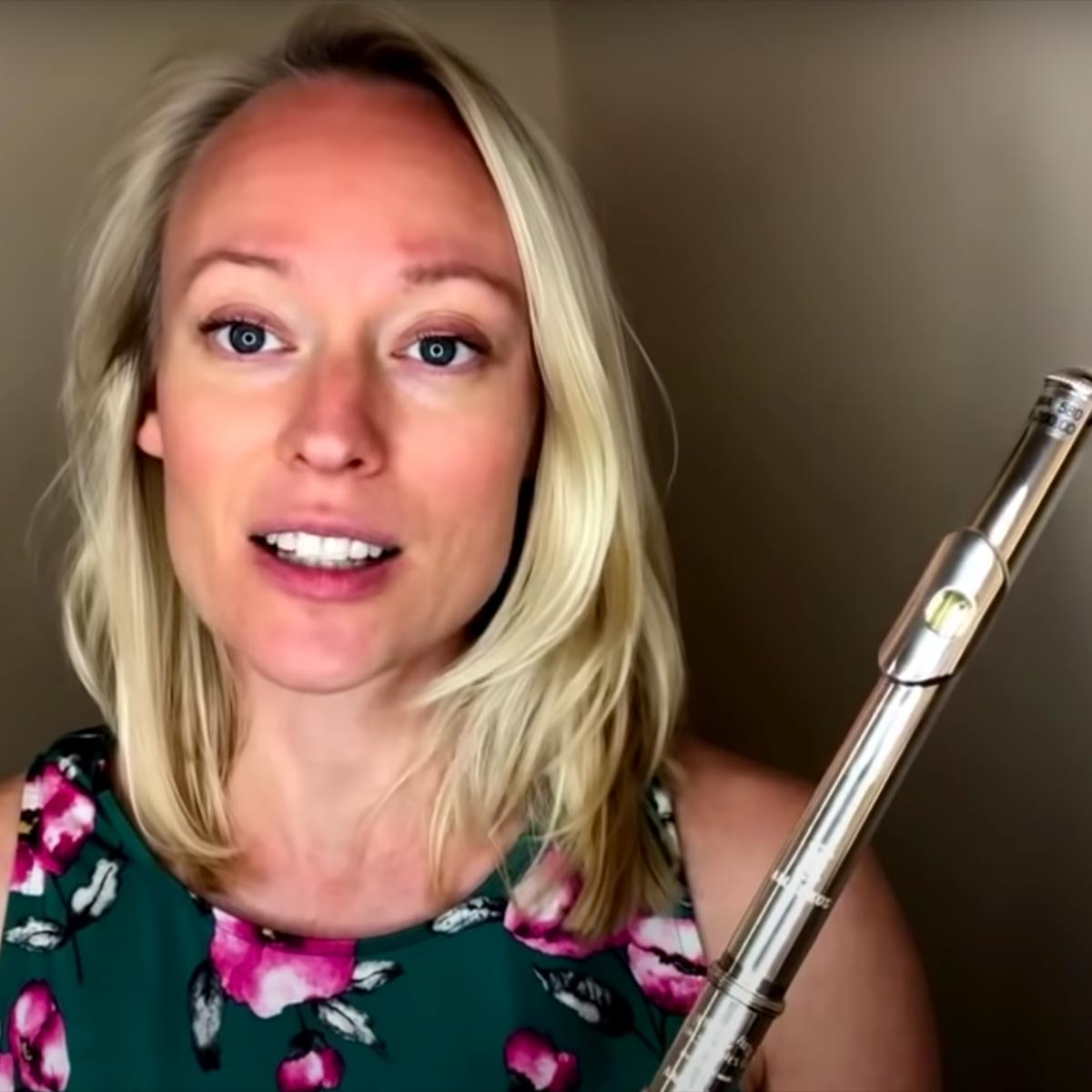# Bevani

1 Music Sheets

• ###### Genres:

A classically trained music artist.

## Artist's Music Sheets

•v B b [zo] d h j J j h d D h J Z c J h Z c P d g J l J g d s D Z l z h D l [zo] J [zh] v B d P d [sb] D h l b f h v d G j z C w y [oh] y J y P j [yd] I p d f d G d T o P D g D P D G y i P s P d P t T D o d o T s [dw] y o s P o y 0 [ts] T o P I y e p [wo] 0 y o p P d h s D [vh] l v h D b [ov] d [zhv] d j J j h s D [vh] l v h D b [ov]
Level: 5
Intermediate